# Cylindrical Tearing Mode Equation

The magnetic structure of a tearing perturbation is determined by the so-called cylindrical tearing mode equation, (3.35), which can be written in the form [4,14]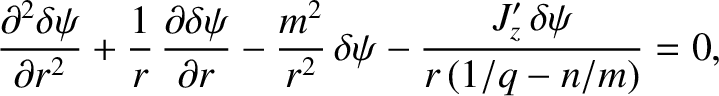(3.60)

where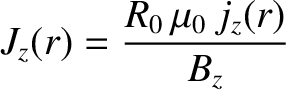(3.61)

is a dimensionless measure of the toroidal current density profile. Note that Equation (3.60) is singular at the so-called rational magnetic flux-surface, radius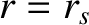, at which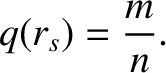(3.62)

At the rational surface,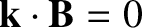, where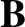is the equilibrium magnetic field, and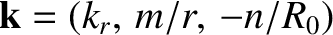the wavevector of the tearing perturbation.